Tags Math

The well-ordering principle states:

The well-ordering principle: Any nonempty set of nonnegative integers has a smallest element.

DUH, you don't say! - seems obvious, doesn't it? This principle is, nevertheless, a very important and fundamental tool for proving other basic principles of number theory.

Consider, for instance, the Division Algorithm:

The Division Algorithm: If m and n are integers with n > 0, then there exist integers q and r, with 0 <= r < n, such that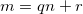.

Again, this is so basic that one may doubt whether it should even be proved. But the well-ordering principle allows us, in fact, to prove the division algorithm in a rigorous manner:

Let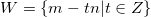. It is obvious that W contains nonnegative integers. Let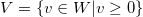. By the well-ordering principle, V has a smallest element, which we'll call r.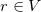, so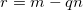for some q and r >= 0 (by the definition of sets W and V, correspondingly).

Now, what's left to prove is that r < n. Let's assume the opposite, namely that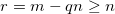. Rearranging: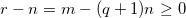. By the definition of V,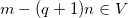(since it has the form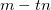for some integer t and is nonnegative). But recall that we called r the smallest element of V, and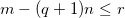, so we have a contradiction.

Therefore, we see that r < n. This completes the proof. Q.E.D.